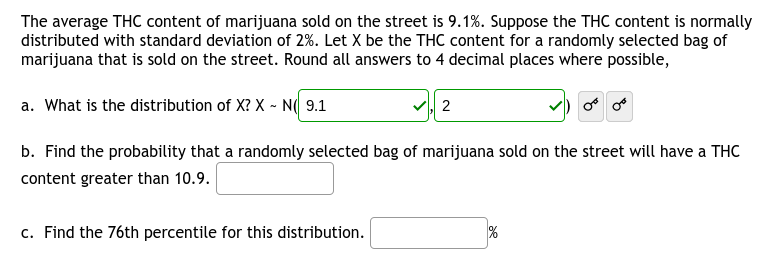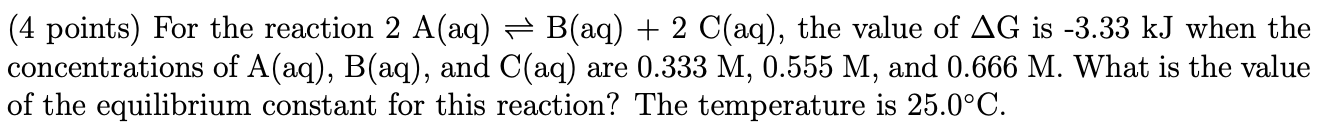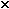Similar Homework Help Questions

The strength of one's immune system can be evaluated in several ways. One of the most popular methods involves measuring the concentration of adenosine triphosphate(ATP) in the blood. In women, the mean blood ATP level is 390 ng/mL, and the standard deviation is 115 ng/mL.It has been hypothesized that women with Major Depressive Disorder (MDD) have decreased immune function. A recent study found that a random sample of 96 women with MDDhad an average blood ATP level of 355 ng/mL.Using...

You are given 40X stock of NaCl, and a10Xstock of SDS, andare asked to make 300mL of a solution that is 1X Nacl and 1X SDS.Outline how you would do this.

Find the net gravitational force exerted by the Earth and themoon on a spaceship with mass 35700 kg located halfway betweenthem.The answer range is 200 and 500 N. I'm not sure how to dothis, I am lost/ HELP!!!!

Evaluate: lim?(x?0)??(cscx-1/x) ?

I am not sure where to start I feel like I am missinginformation.Assume that the earth has an average surface temperature of22°C and is a perfect emitter ofradiation (e = 1). Find the energy per second per squaremeterthat the earth radiates into space.Please help me with this. The answer key is 8.51. I am lost. Thank you so much! (4 points) For the reaction 2 A(aq) = B(aq) + 2 C(aq), the value of AG is -3.33 kJ when the concentrations of A(aq), B(aq), and C(aq) are 0.333 M, 0.555 M, and 0.666 M. What is the value of the equilibrium constant for this reaction? The temperature is 25.0°C. Show transcribed image text

A sample of 10.1 g ammonium nitrate, NH4NO3, was dissolved in 125.0 g of water in a coffee-cup calorimeter. The temperature changed from 24.5 deg C to 18.8 deg C.Calculate the heat of solution (i.e., delta H of solution)of ammonium nitrate in kJ/mol. Assume that the energy exchange involved only the solution and that thespecific heat of the solution is 4.18 J/g. deg. C. Show work or include as much explanation as needed for this calculation. If you give just...

. The following data are from a two-factor study examining the effects of three treatment conditions on males and females.a. Use an ANOVA with a= .05 for all tests to evaluate the significance of the main effects and the interaction.b. Calculate the n2 values to measure the effect size for the two main effects and for the interaction.TreatmentsI II III1 7 92 2 11Male M=3 m=6 m=9T=9 t=18 t=27 T male=54Ss=14 SS=26 SS=8Factor A:GenderFemale3 10 16 N = 181 11...If R = [1, 5] [0, 2], usea Riemann sum with m = 4, n = 2 to estimatethevalue of the following. Take the sample points to be the upper leftcorners of the subrectangles.I tried this problem numerous times and can not figure it out. Mybook does not help hopefully someone on here can help me. Thankyou.